### 东风悦达起亚 起亚KX3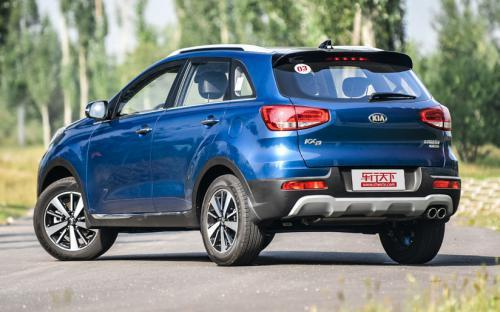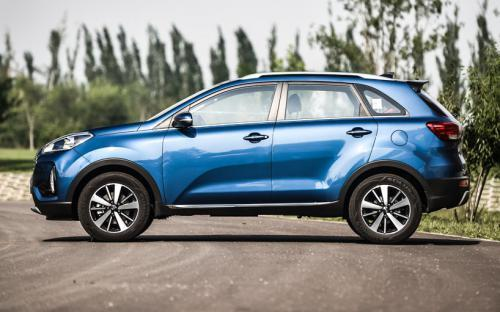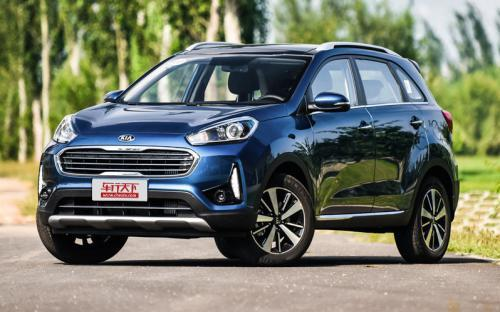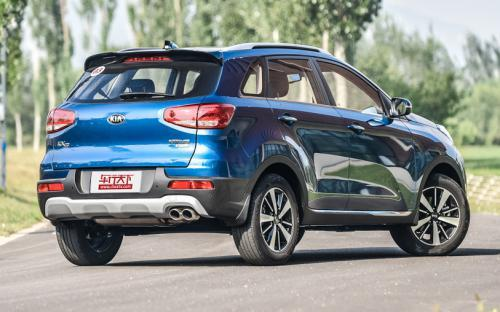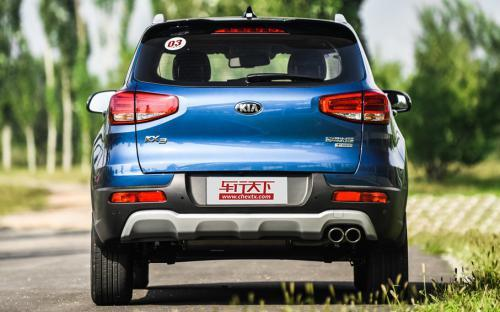0 种颜色可选2017款最低售价：11.68 万元起

4270(mm)1780(mm)1630(mm)##### 配置亮点：
• 胎压监测装置

• ISOFIX儿童座椅接口

• 车身稳定控制(ESC/ESP/DSC等)

• 电动天窗

• 定速巡航

• 后倒车雷达

• 真皮座椅

• GPS导航系统

• 氙气大灯

• 后视镜加热

• 提交
2017款 1.6L 自动傲雅版 (119张)
• 2017款 1.6L 自动傲雅版 (119张)
• 2017款 1.6T 自动傲尊版 (184张)
• 东风悦达起亚 起亚KX3 绕车实拍• 东风悦达起亚 起亚KX3 在售车型

排量 车型 厂商指导价 本地最低报价 购车工具
1.6L
11.68万
11.68万

1.6L 自动傲风版 6挡手自一体
12.68万
1.6L 自动傲雅版 6挡手自一体
14.08万
14.08万

1.6L 自动傲尊版 6挡手自一体
15.48万
2.0L
11.68万
11.68万

1.6L 自动傲风版 6挡手自一体
12.68万
1.6L 自动傲雅版 6挡手自一体
14.08万
14.08万

1.6L 自动傲尊版 6挡手自一体
15.48万
1.6T
1.6T 自动傲雅版 7挡双离合
15.28万
1.6T 自动傲尊版 7挡双离合
17.78万
17.78万

东风悦达起亚 起亚KX3 经销商

查看更多 >>

### 东风悦达起亚 起亚KX3 动力加速

起亚KX3 0-100公里加速时间分布在 0.0-秒 属于 超跑级

动力级别 加速时间 车型

东风悦达起亚 起亚KX3 视频

东风悦达起亚 起亚KX3 新闻资讯

# 全新一代傲跑燃爆搜狐时尚盛典

国产新车 超过11997次关注

12月18日,中国时尚界的“奥斯卡”——2019年搜狐时尚盛典在北京隆重举行,东风悦达起亚作为本次时尚盛典的总赞助商,由“IN核SUV”全新一代傲跑携手搜狐共启时尚之旅...

# 引以为傲 这款小型SUV市场搅局

国产新车 超过14768次关注

颜值出众\配置过硬\这款小型SUV将成为市场搅局者？没错，东风悦达起亚全新一代傲跑正式上市,共推4款车型,售价为10.88万元-12.58万元。东风悦达起亚全国首推“包牌价...

# 全国首推包牌价 全新一代傲跑上市

国产新车 超过9101次关注

新一代傲跑再次刷新东风悦达起亚智能化、年轻化的品牌形象。东风悦达起亚IN核SUV——全新一代傲跑在广州车展正式上市,共推出四款车型,售价区间为10.88万元-12.58万...

# 换脸重新来战 起亚KX3售11.68-17.78万

国产新车 超过9412次关注

2016年9月19日，东风悦达起亚新款KX3正式上市。新车搭载了1.6L和1.6T发动机，匹配手动、自动和双离合变速箱。根据不同配置，新车共推出6款车型，售价区间为11.68-17.78万。

# 不可小看 起亚小型SUV“傲跑”评测

试驾评测 超过19284次关注

傲跑来了！似乎韩系车慢了半拍，难道是为了战略求稳！北京现代ix25的推出并且表现不俗，起亚才慢步跟上，二者默契有余的同时小型SUV的竞争又多了一位生力军！

猜你喜欢

﻿
• 快速找车
• 选择品牌
• 选择品牌
• A  奥迪
• A  阿斯顿·马丁
• A  阿尔法·罗密欧
• B  宝沃
• B  布加迪
• B  巴博斯
• B  保时捷
• B  宾利
• B  奔驰
• B  宝马
• B  本田
• B  别克
• B  标致
• B  比亚迪
• B  宝骏
• B  北汽制造
• B  北汽新能源
• B  北汽幻速
• B  北汽威旺
• B  北京汽车
• B  奔腾
• B  北汽绅宝
• C  长安
• C  长安商用
• C  长城
• C  昌河
• D  大众
• D  道奇
• D  DS
• D  东南
• D  东风风神
• D  东风风行
• D  东风小康
• D  东风风度
• D  东风
• F  福特
• F  丰田
• F  菲亚特
• F  法拉利
• F  福田
• F  福迪
• F  福汽启腾
• G  观致
• G  广汽传祺
• G  广汽吉奥
• G  GMC
• H  红旗
• H  汉腾汽车
• H  哈弗
• H  哈飞
• H  海格
• H  海马
• H  华颂
• H  黄海
• H  华泰
• H  恒天
• J  吉利汽车
• J  捷豹
• J  Jeep
• J  江淮
• J  江铃
• J  金杯
• J  九龙
• J  金旅
• K  凯翼
• K  凯迪拉克
• K  克莱斯勒
• K  科尼塞克
• K  卡威
• K  开瑞
• L  路虎
• L  林肯
• L  劳斯莱斯
• L  兰博基尼
• L  雷克萨斯
• L  铃木
• L  雷诺
• L  理念
• L  力帆
• L  莲花汽车
• L  猎豹
• L  路特斯
• L  陆风
• M  马自达
• M  MG
• M  MINI
• M  玛莎拉蒂
• M  摩根
• M  迈凯轮
• N  纳智捷
• O  欧宝
• O  讴歌
• O  欧朗
• Q  奇瑞
• Q  起亚
• Q  启辰
• R  日产
• R  荣威
• R  瑞麒
• S  三菱
• S  斯威汽车
• S  萨博
• S  smart
• S  斯柯达
• S  斯巴鲁
• S  思铭
• S  双龙
• S  上汽大通
• S  双环
• T  特斯拉
• T  腾势
• W  沃尔沃
• W  五菱汽车
• W  五十铃
• W  威兹曼
• W  威麟
• X  现代
• X  雪佛兰
• X  雪铁龙
• X  西雅特
• Y  一汽
• Y  英菲尼迪
• Y  英致
• Y  依维柯
• Y  野马汽车
• Y  永源
• Z  众泰
• Z  中华
• Z  中兴
• Z  知豆
• 选择车系
• 选择车系
• 车型对比
• 选择品牌
• 选择品牌
• A  奥迪
• A  阿斯顿·马丁
• A  阿尔法·罗密欧
• B  宝沃
• B  布加迪
• B  巴博斯
• B  保时捷
• B  宾利
• B  奔驰
• B  宝马
• B  本田
• B  别克
• B  标致
• B  比亚迪
• B  宝骏
• B  北汽制造
• B  北汽新能源
• B  北汽幻速
• B  北汽威旺
• B  北京汽车
• B  奔腾
• B  北汽绅宝
• C  长安
• C  长安商用
• C  长城
• C  昌河
• D  大众
• D  道奇
• D  DS
• D  东南
• D  东风风神
• D  东风风行
• D  东风小康
• D  东风风度
• D  东风
• F  福特
• F  丰田
• F  菲亚特
• F  法拉利
• F  福田
• F  福迪
• F  福汽启腾
• G  观致
• G  广汽传祺
• G  广汽吉奥
• G  GMC
• H  红旗
• H  汉腾汽车
• H  哈弗
• H  哈飞
• H  海格
• H  海马
• H  华颂
• H  黄海
• H  华泰
• H  恒天
• J  吉利汽车
• J  捷豹
• J  Jeep
• J  江淮
• J  江铃
• J  金杯
• J  九龙
• J  金旅
• K  凯翼
• K  凯迪拉克
• K  克莱斯勒
• K  科尼塞克
• K  卡威
• K  开瑞
• L  路虎
• L  林肯
• L  劳斯莱斯
• L  兰博基尼
• L  雷克萨斯
• L  铃木
• L  雷诺
• L  理念
• L  力帆
• L  莲花汽车
• L  猎豹
• L  路特斯
• L  陆风
• M  马自达
• M  MG
• M  MINI
• M  玛莎拉蒂
• M  摩根
• M  迈凯轮
• N  纳智捷
• O  欧宝
• O  讴歌
• O  欧朗
• Q  奇瑞
• Q  起亚
• Q  启辰
• R  日产
• R  荣威
• R  瑞麒
• S  三菱
• S  斯威汽车
• S  萨博
• S  smart
• S  斯柯达
• S  斯巴鲁
• S  思铭
• S  双龙
• S  上汽大通
• S  双环
• T  特斯拉
• T  腾势
• W  沃尔沃
• W  五菱汽车
• W  五十铃
• W  威兹曼
• W  威麟
• X  现代
• X  雪佛兰
• X  雪铁龙
• X  西雅特
• Y  一汽
• Y  英菲尼迪
• Y  英致
• Y  依维柯
• Y  野马汽车
• Y  永源
• Z  众泰
• Z  中华
• Z  中兴
• Z  知豆
• 选择车系
• 选择车系
• 选择车型
• 选择车型
• 意见反馈